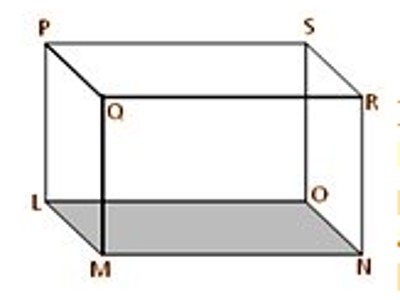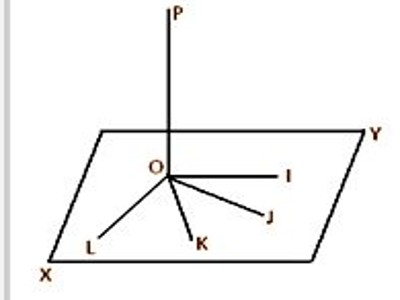A curved surface is considered a large set of points. Every point in the curve has two neighbors excluding the endpoints. Plane Surface is a flat surface in which a straight line joining any two of its points lies entirely on that surface. It’s a bit difficult to visualize a plane because, in real life, there is nothing that we can use as a true example of a geometric plane.

A surface is called curved surface when it is not a plane surface.

(i) Lines or points are said to be co-planar if they lie on the same plane; in other words, lines or points are co-planar if a plane can be made to pass through them.

(ii) Two co-planar straight lines are either parallel or they intersect at a point. Two straight lines are said to be parallel when they are co-planar and they do not meet however indefinitely they are produced in both directions.

(iii) Two straight lines are said to be skew (or non-coplanar) if a plane cannot be made to pass through them. In other words, two straight lines are said to be skew when they do not meet at a point and they are not parallel.

(iv) Two planes are said to be parallel if they do not meet when extended infinitely in all directions.

(v) A straight line is said to be parallel to a plane if they do not meet when both are produced infinitely.In the given picture we observe that the lines LM, MN, NO, and OL lie in the plane LMNO, that is, they are co-planar. The lines LM and LO meet at L and the lines LM and ON are parallel. LP and MN are skew lines and the line QR is parallel to the plane LPSO. The planes ABFE and DCGH are parallel.

A straight line is said to be perpendicular to a plane if it is perpendicular to every straight lines drawn in the plane through the point where the line meets the plane.A straight line perpendicular to a plane is called a normal to the plane. In the given figure, the straight line OP meets the plane XY at O; if OP is perpendicular to every straight lines OI, OJ, OK, OD etc. drawn through O in the XY plane then OP is perpendicular (or a normal) to the plane XY.

A straight line parallels to the direction of a plumb-line hanging freely at rest is called a vertical line. A plane which is perpendicular to a vertical line is called the horizontal plane. A straight line drawn in a horizontal plane is called a horizontal line.

Information Source: